# Non-Linear Diffusion Flows¶

Important: Please read the installation page for details about how to install the toolboxes. $\newcommand{\dotp}{\langle #1, #2 \rangle}$ $\newcommand{\enscond}{\lbrace #1, #2 \rbrace}$ $\newcommand{\pd}{ \frac{ \partial #1}{\partial #2} }$ $\newcommand{\umin}{\underset{#1}{\min}\;}$ $\newcommand{\umax}{\underset{#1}{\max}\;}$ $\newcommand{\umin}{\underset{#1}{\min}\;}$ $\newcommand{\uargmin}{\underset{#1}{argmin}\;}$ $\newcommand{\norm}{\|#1\|}$ $\newcommand{\abs}{\left|#1\right|}$ $\newcommand{\choice}{ \left\{ \begin{array}{l} #1 \end{array} \right. }$ $\newcommand{\pa}{\left(#1\right)}$ $\newcommand{\diag}{{diag}\left( #1 \right)}$ $\newcommand{\qandq}{\quad\text{and}\quad}$ $\newcommand{\qwhereq}{\quad\text{where}\quad}$ $\newcommand{\qifq}{ \quad \text{if} \quad }$ $\newcommand{\qarrq}{ \quad \Longrightarrow \quad }$ $\newcommand{\ZZ}{\mathbb{Z}}$ $\newcommand{\CC}{\mathbb{C}}$ $\newcommand{\RR}{\mathbb{R}}$ $\newcommand{\EE}{\mathbb{E}}$ $\newcommand{\Zz}{\mathcal{Z}}$ $\newcommand{\Ww}{\mathcal{W}}$ $\newcommand{\Vv}{\mathcal{V}}$ $\newcommand{\Nn}{\mathcal{N}}$ $\newcommand{\NN}{\mathcal{N}}$ $\newcommand{\Hh}{\mathcal{H}}$ $\newcommand{\Bb}{\mathcal{B}}$ $\newcommand{\Ee}{\mathcal{E}}$ $\newcommand{\Cc}{\mathcal{C}}$ $\newcommand{\Gg}{\mathcal{G}}$ $\newcommand{\Ss}{\mathcal{S}}$ $\newcommand{\Pp}{\mathcal{P}}$ $\newcommand{\Ff}{\mathcal{F}}$ $\newcommand{\Xx}{\mathcal{X}}$ $\newcommand{\Mm}{\mathcal{M}}$ $\newcommand{\Ii}{\mathcal{I}}$ $\newcommand{\Dd}{\mathcal{D}}$ $\newcommand{\Ll}{\mathcal{L}}$ $\newcommand{\Tt}{\mathcal{T}}$ $\newcommand{\si}{\sigma}$ $\newcommand{\al}{\alpha}$ $\newcommand{\la}{\lambda}$ $\newcommand{\ga}{\gamma}$ $\newcommand{\Ga}{\Gamma}$ $\newcommand{\La}{\Lambda}$ $\newcommand{\si}{\sigma}$ $\newcommand{\Si}{\Sigma}$ $\newcommand{\be}{\beta}$ $\newcommand{\de}{\delta}$ $\newcommand{\De}{\Delta}$ $\newcommand{\phi}{\varphi}$ $\newcommand{\th}{\theta}$ $\newcommand{\om}{\omega}$ $\newcommand{\Om}{\Omega}$

This tours details non-linear diffusion PDEs. A good reference for diffusion flows in image processing is Weickert98.

In :
addpath('toolbox_signal')


## Non-linear Second-order Parabolic PDEs¶

This tour defines PDE flows that are non-linear extension of the heat equation. Non-linearity is crucial to produce edge-aware flows that do not blur the edges.

It is also important to produce contrast invariant and affine invariant flows.

These flows can be written as $$\pd{f_t}{t}(x) = G(\nabla f_t(x), \nabla^2 f_t(x)).$$ with $f_0$ defined at time $t=0$ (whith the slight modification that a blurring is introduced in the Perona-Malick flow to stabilize it).

They are discretized in space by considering a discrete image of $N = n \times n$ pixels.

In :
n = 256;


We use finite difference operators $\nabla$ and $\text{div}=-\nabla^*$ with periodic boundary conditions.

Load an image $f_0 \in \RR^N$, that will be used to initialize the flow at time $t=0$.

In :
name = 'hibiscus';
f0 = rescale( sum(f0,3) );


Display it.

In :
clf;
imageplot(f0);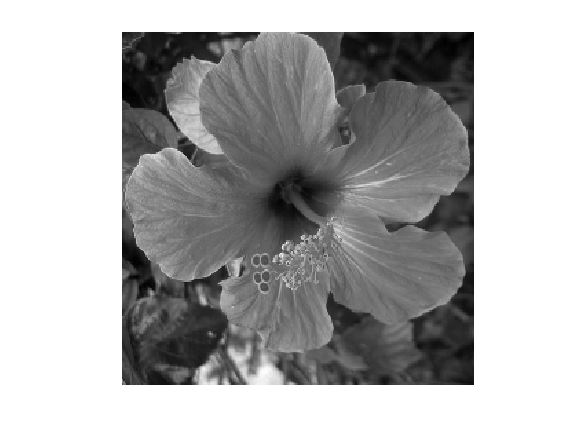The flow is discretized in time using an explicit time-stepping $$f^{(\ell+1)}(x) = f^{(\ell)}(x) • \tau G(\nabla f^{(\ell)}(x), \nabla^2 f^{(\ell)}(x)).$$ Here $\tau>0$ should be small enough, and $f^{(\ell)}$ produces an approximation of $f_t$ at time $t=\ell\tau$.

Convolutions can be computed in $O(N\log(N))$ operations using the FFT, since $$g = f \star h \qarrq \forall \om, \quad \hat g(\om) = \hat f(\om) \hat h(\om).$$

In :
cconv = @(f,h)real(ifft2(fft2(f).*fft2(h)));


Define a Gaussian blurring kernel of width $\si$: $$h_\si(x) = \frac{1}{Z} e^{ -\frac{x_1^2+x_2^2}{2\si^2} }$$ where $Z$ ensures that $\hat h_\si(0)=1$.

In :
t = [0:n/2 -n/2+1:-1];
[X2,X1] = meshgrid(t,t);
normalize = @(h)h/sum(h(:));
h = @(sigma)normalize( exp( -(X1.^2+X2.^2)/(2*sigma^2) ) );


Set the value of $\sigma>0$.

In :
sigma = .5;


Define blurring operator.

In :
blur = @(f)cconv(f,h(sigma));


## Perona-Malik Flow¶

The Perona-Malik non-linear diffusion is defined as $$\pd{f_t}{t} = \text{div}\pa{ g_\la( \norm{\nabla f_t^\si} ) \nabla f_t }$$ where $f^\si = f \star h_\si$.

This PDE was introduced in PerMal90.

Here, $g_\la : \RR^+ \rightarrow \RR^+$ is a non-increasing function, that wechose in the following as $$g_\la(s) = \frac{1}{\sqrt{1 + (s/\la)^2}}.$$

In :
g = @(s,lambda)1./sqrt( 1+(s/lambda).^2 );


Note that in the limit $\la \rightarrow +\infty$, one recovers the linear heat equation $$\pd{f_t}{t} = \Delta f_t$$ where $\Delta=\text{div} \circ \nabla$ is the Laplacian.

Define $A(f) = \norm{\nabla f^\si}$.

In :
amplitude = @(u)repmat( sqrt( sum(u.^2,3) ), [1 1 2]);


Initialize the solution at time $t=0$.

In :
f = f0;


Set the value of $\lambda$.

In :
lambda = .01;


Set the value of the descent step size $\tau>0$.

In :
tau = .2;


Perform one time stepping.

In :
f = f + tau * div( g(A(f),lambda) .* grad(f) );


Final time.

In :
T = .5/lambda;


Number of iteration to reach this final time.

In :
niter = ceil(T/tau);


Exercise 1

Implement the Perona-Malick diffusion flow for $\la = 10^{-2}$.

In :
exo1()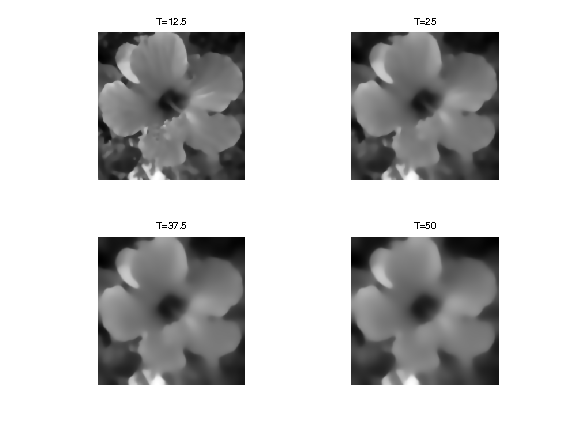In :
%% Insert your code here.


Exercise 2

Implement the Perona-Malick diffusion flow for $\la = 10^{-3}$.

In :
exo2()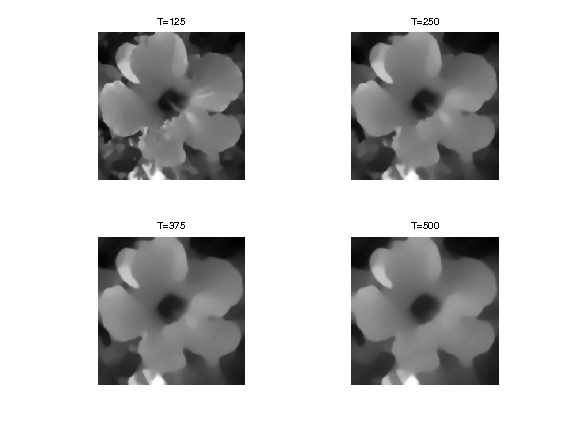In :
%% Insert your code here.


## Mean Curvature Flow¶

In the limit that $\la \rightarrow +\infty$ and $\si \rightarrow 0$ the Perona-Malick flow becomes $$\pd{f_t}{t} = \text{curv}(f_t)$$ where $$\text{curv}(f) = \text{div}\pa{ \frac{\nabla f}{\norm{\nabla f}} }.$$ One can show that $\text{curv}(f)(x)$ is the curvature at location $x$ of the level set $\enscond{y}{f(y)=f(x)}$.

This flow is the gradient descent flow associated to the total variation $$J(f) = \int_{\RR^2} \norm{\nabla f(x)} d x,$$ which can be extended to non-smooth functions of bounded variations. Indeed, a (sub)gradient of $J$ is $-\text{curv}(f)$.

Total variation regularization was introcued in

A closely related flow is the so-called mean curvature flow $$\pd{f_t}{t} = \norm{\nabla f_t} \text{curv}(f_t).$$ One can show that this flow is contrast-invariant. This means that for any non-decreasing function $\phi : \RR \rightarrow \RR$, $\phi \circ f_t$ is also a solution of the PDE (possibly up to a re-parameterization of the time variable).

One can show that any contrast-invariant flow can be written as $$\pd{f_t}{t} = \norm{\nabla f_t} \psi( \text{curv}(f_t) )$$ for a non-decreasing function $\psi : \RR \rightarrow \RR$.

Implement the curv operator. We use a small $\epsilon$ to avoid division by 0.

In :
epsilon = 1e-6;
amplitude = @(u)sqrt(sum(u.^2,3)+epsilon^2);
normalize = @(u)u./repmat( amplitude(u), [1 1 2]);


Exercise 3

Implement the mean curvature flow.

In :
exo3()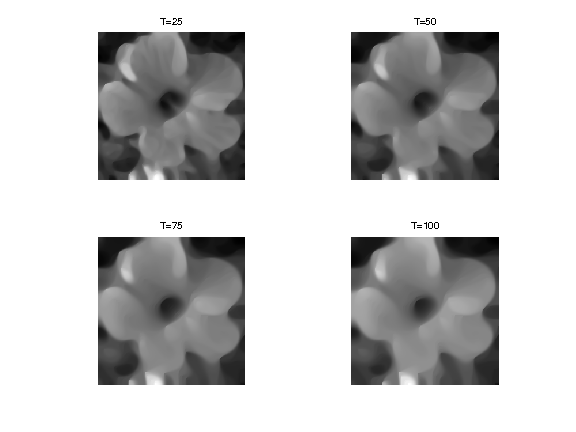In :
%% Insert your code here.


## Affine Invariant Flow¶

A flow is affine invariant if $f_t \circ A$ is also a solution of the PDE (possibly up to a re-parameterization of the time variable).

The only affine invariant and contrast invariant flow is $$\pd{f_t}{t} = \norm{\nabla f_t} \text{curv}(f_t)^{1/3}.$$ where $s^{1/3}= \text{sign}(s) \abs{s}^{1/3}$.

This result was discovered independently in AlvGuiLiMo93 and SapTann93

Exercise 4

Implement the affine-invariant curvature flow.

In :
exo4()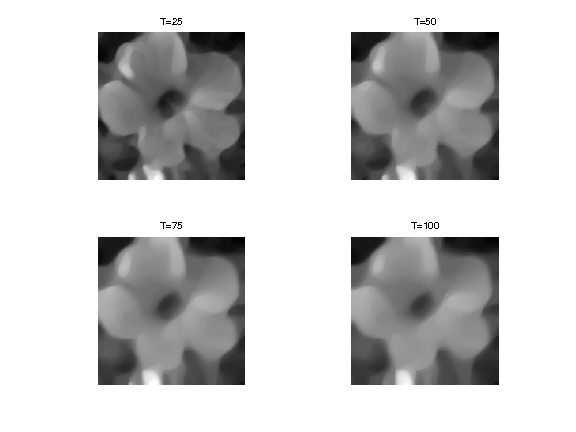In :
%% Insert your code here.